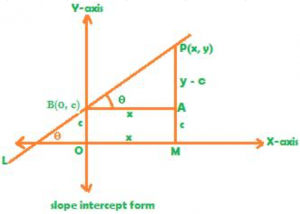Slope intercept form of equation of a straight line is y = mx + c, in which, m is slope of the straight line and c is the intercept, i.e. the y intercept specifically. To understand in more detail the equation of a straight line in slope intercept form, consider the following figure:In the above figure, the straight line L makes angle θ with the x-axis in positive direction, i.e. anti-clockwise direction. Also, the straight line L passes through the point B (0, c).

In the point B (0, c), the y coordinate is the y intercept of the line L. {The straight line L is also passing through the point P(x, y)}. By definition, y intercept is the y coordinate in a point on the y axis through which the straight line L passes. It is cin the above figure.

Now, in triangle ABP, tan θ = (PA/BA) = (y – c /x), but tan θ is slope of the straight line L, because, by definition, slope of a straight line L which makes angle θ with the positive direction of the x-axis in anti-clockwise direction is tan θ .

Now substitute m in tan θ, as slope of a line is conventionally denoted by the letter m. So, m = (y – c /x), i.e. y – c = mx, so, finally y = mx + c.  This is the equation of a straight line L in slope intercept form, in which m is the slope of the line and c is the y intercept.

## Example 1:

Use slope intercept form to find the equation of a straight line L whose slope is 2 and which passes through the point P (0, 3)

### Solution:

Recall, the formula for slope intercept form of equation of a straight line L is y = mx + c from the question, slope is 2 and y intercept is 3. Y intercept is 3 because the point P (0, 3) lies on the y axis, first of all, and secondly, the straight line L passes through this point, because of which the y coordinate 3 turns into y intercept of the line L.

So, 3 can be written in c in the above slope intercept form of the equation. So, y = 2x + 3 is the equation of the required straight line in slope intercept form.

## Example 2:

Find the equation of a straight line L having slope 2 and passing through the point P (3, 0)

### Solution:

Read carefully that the point P (3, 0) lies on x axis. So, you are not given the y intercept; rather the x intercept is given. That means, the y intercept needs to be found, as follows:

To find the y intercept: substitute 0 in y; 2 in m; and 3 in x in the equation for slope intercept form y = mx + c, as below 0 = 2 × 3 + c, c = -6. Now plug in the values of slope and y intercept in m and c respectively to finally find the equation of the given straight line L in slope intercept form: y = 2x – 6